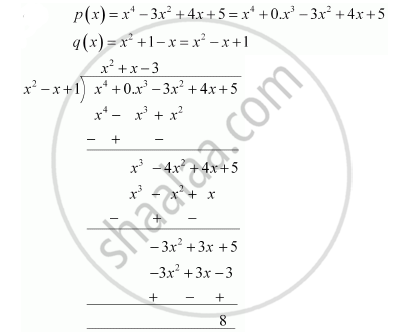Share

Books Shortlist

# Divide the polynomial p(x) by the polynomial g(x) and find the quotient and remainder in each of the following :  p(x) = x^4 – 3x^2 + 4x + 5,   g(x) = x^2 + 1 – x - CBSE Class 10 - Mathematics

ConceptDivision Algorithm for Polynomials

#### Question

Divide the polynomial p(x) by the polynomial g(x) and find the quotient and remainder in each of the following : p(x) = x4 – 3x2 + 4x + 5,   g(x) = x2 + 1 – x

#### SolutionQuotient = x2 + x - 3

Remainder = 8

Is there an error in this question or solution?

#### APPEARS IN

NCERT Solution for Mathematics Textbook for Class 10 (2019 to Current)
Chapter 2: Polynomials
Ex.2.30 | Q: 1.2 | Page no. 36

#### Video TutorialsVIEW ALL 

Solution Divide the polynomial p(x) by the polynomial g(x) and find the quotient and remainder in each of the following :  p(x) = x^4 – 3x^2 + 4x + 5,   g(x) = x^2 + 1 – x Concept: Division Algorithm for Polynomials.
S